# RS Aggarwal Solutions for Class 9 Chapter 6: Introduction to Euclid's Geometry

Euclid’s method was constructed by a Greek Mathematician who postulated the theorems and axioms. The postulates and axioms which were introduced by Euclid are used primarily for arithmetic and measurement. The students while solving problems on a regular basis can make use of the RS Aggarwal Solutions for Class 9 which can boost their problem solving skills.

The solutions are explained in simple language, which is understandable by the students. These solutions are mainly prepared by experts having good knowledge about the concepts to make the exam preparation of the students effective. RS Aggarwal Solutions for Class 9 Chapter 6 Introduction to Euclid’s Geometry are provided here.

## RS Aggarwal Solutions for Class 9 Chapter 6 : Introduction to Euclid’s Geometry Download PDF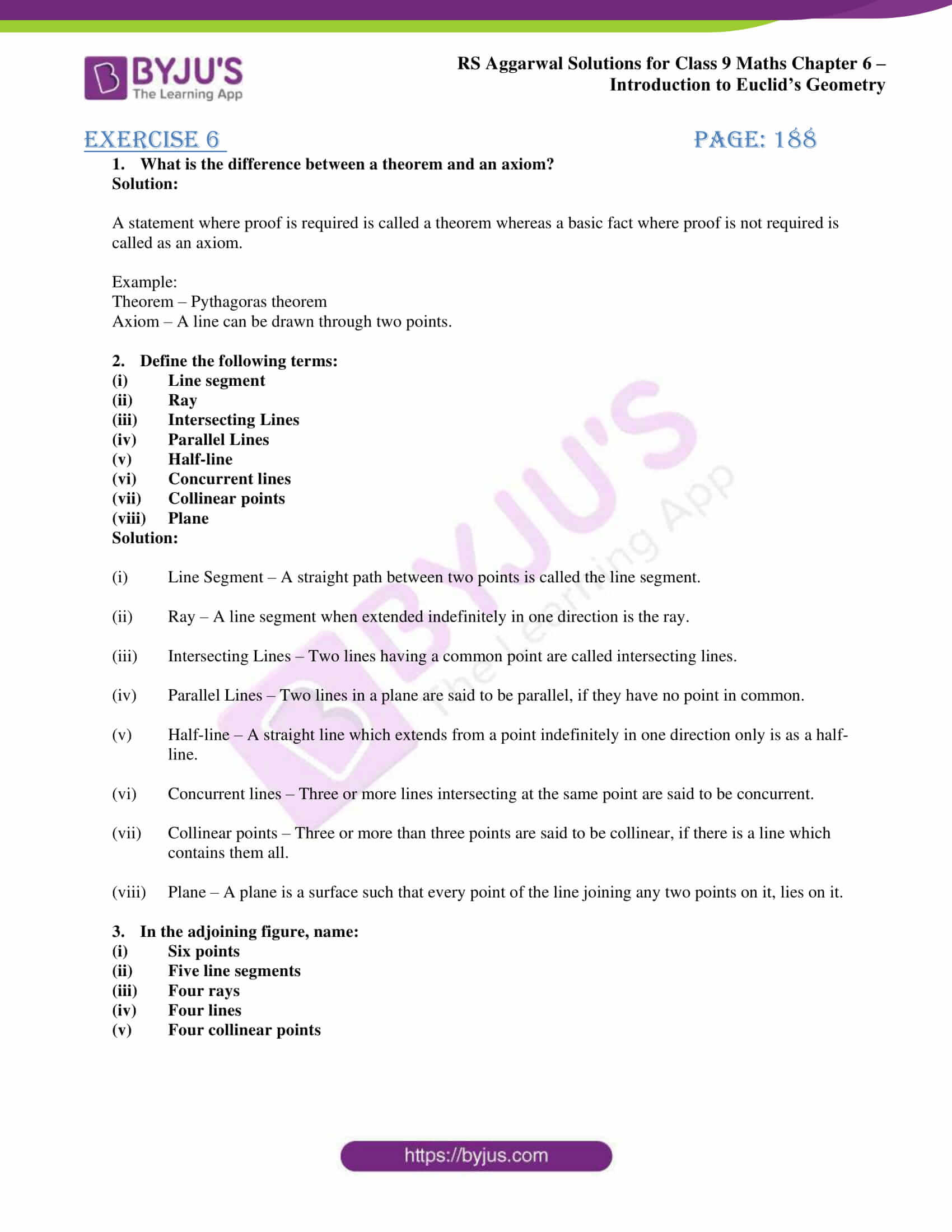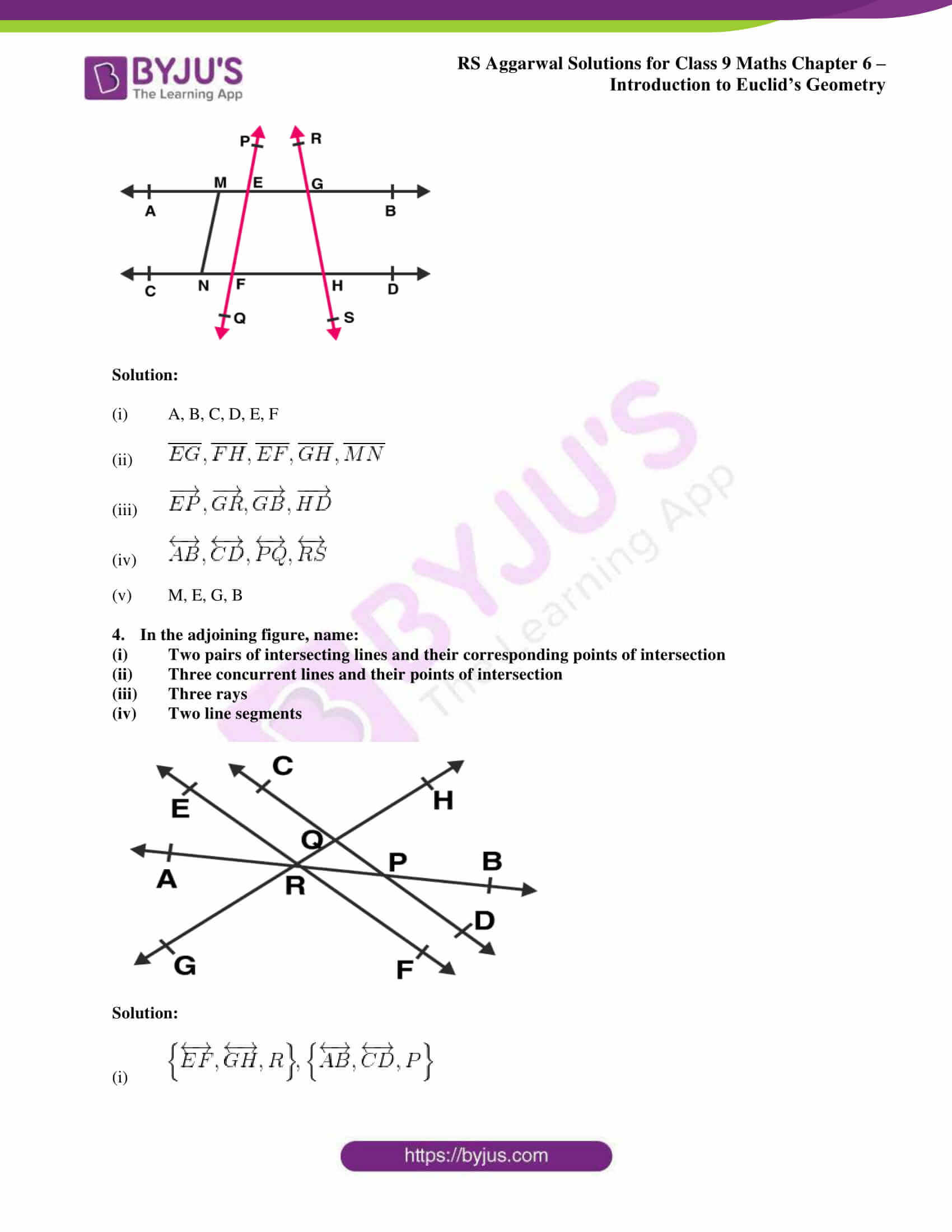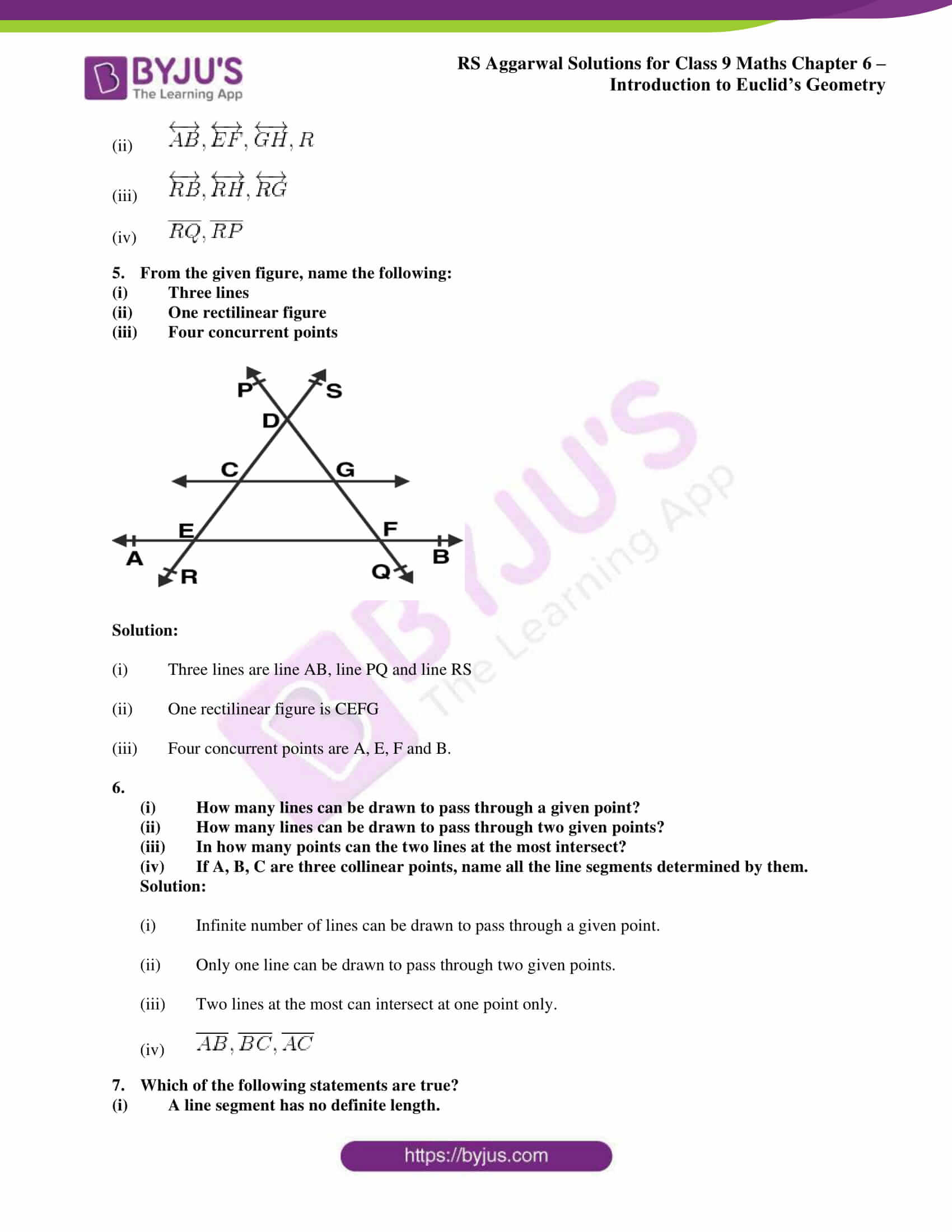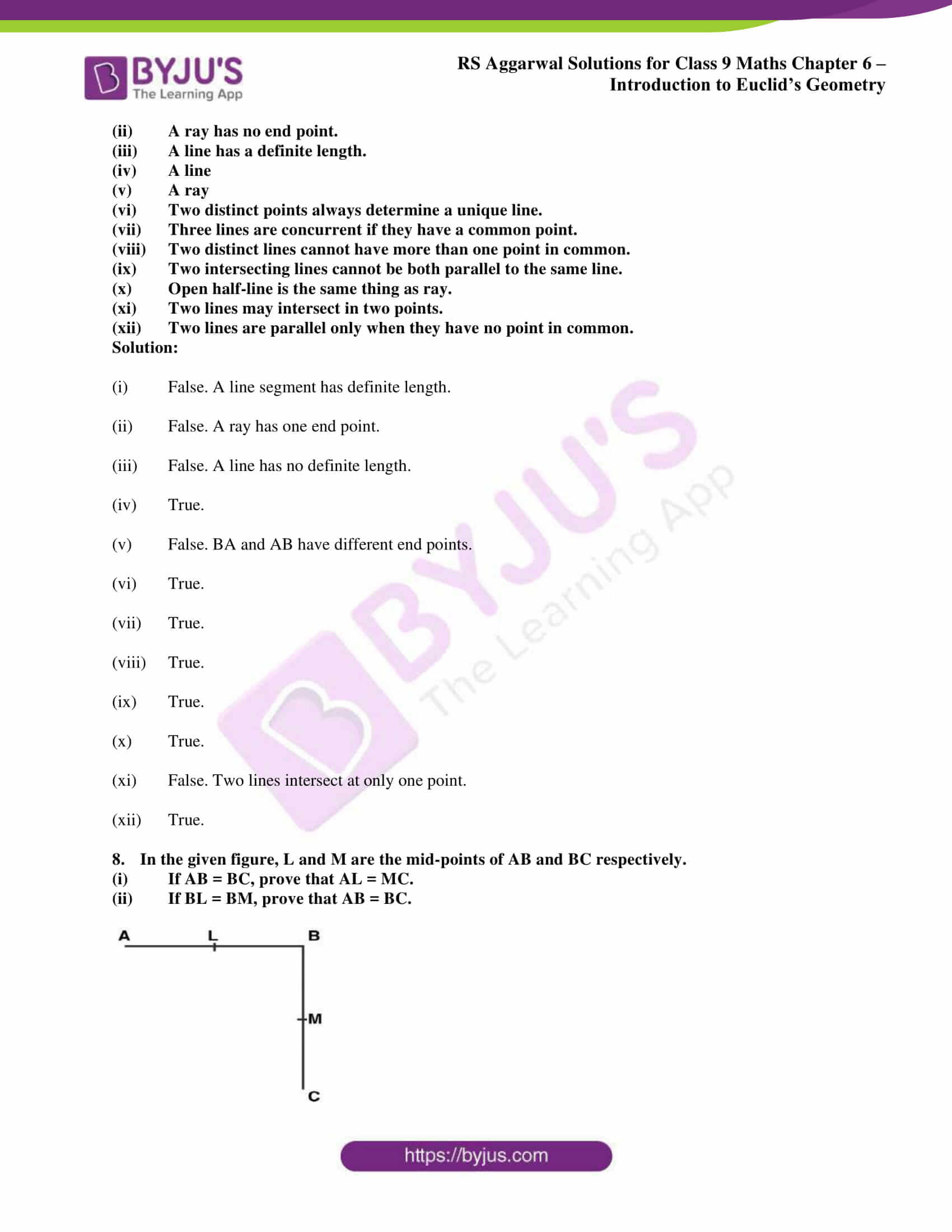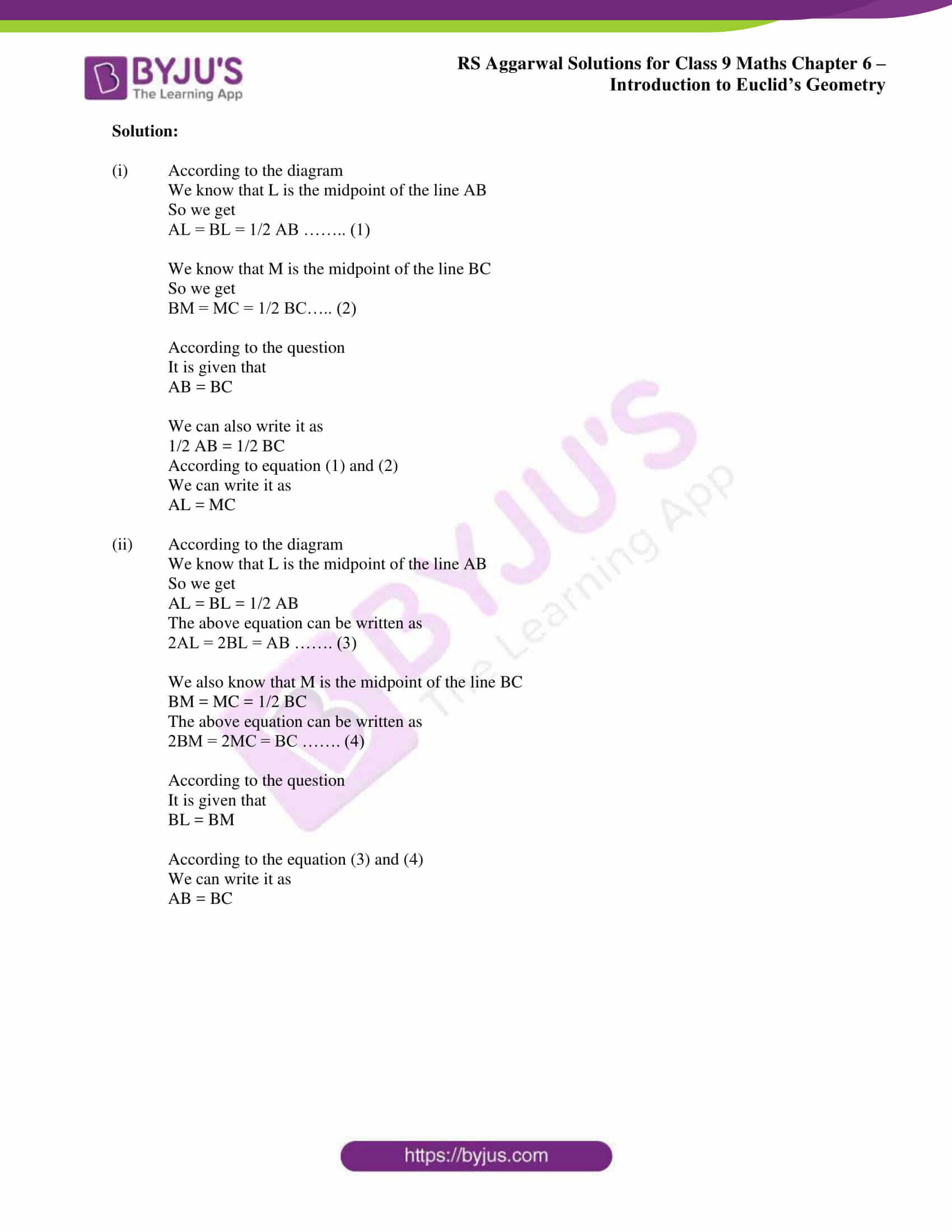## Access RS Aggarwal Solutions for Class 9 Chapter 6: Introduction to Euclid’s Geometry

Exercise 6 PAGE: 188

1. What is the difference between a theorem and an axiom?

Solution:

A statement where proof is required is called a theorem whereas a basic fact where proof is not required is called as an axiom.

Example:

Theorem – Pythagoras theorem

Axiom – A line can be drawn through two points.

2. Define the following terms:

1. Line segment
2. Ray
3. Intersecting Lines
4. Parallel Lines
5. Half-line
6. Concurrent lines
7. Collinear points
8. Plane

Solution:

1. Line Segment – A straight path between two points is called the line segment.
2. Ray – A line segment when extended indefinitely in one direction is the ray.
3. Intersecting Lines – Two lines having a common point are called intersecting lines.
4. Parallel Lines – Two lines in a plane are said to be parallel, if they have no point in common.
5. Half-line – A straight line which extends from a point indefinitely in one direction only is as a half-line.
6. Concurrent lines – Three or more lines intersecting at the same point are said to be concurrent.
7. Collinear points – Three or more than three points are said to be collinear, if there is a line which contains them all.
8. Plane – A plane is a surface such that every point of the line joining any two points on it, lies on it.

3. In the adjoining figure, name:

1. Six points
2. Five line segments
3. Four rays
4. Four lines
5. Four collinear points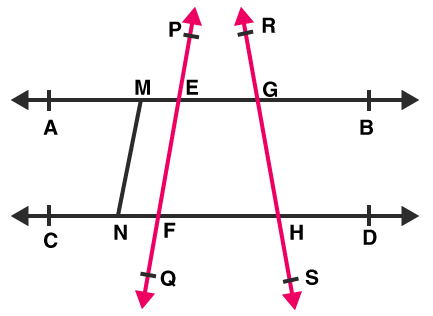Solution:

1. A, B, C, D, E, F
2.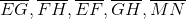3.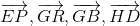4.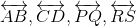5. M, E, G, B

4. In the adjoining figure, name:

1. Two pairs of intersecting lines and their corresponding points of intersection
2. Three concurrent lines and their points of intersection
3. Three rays
4. Two line segments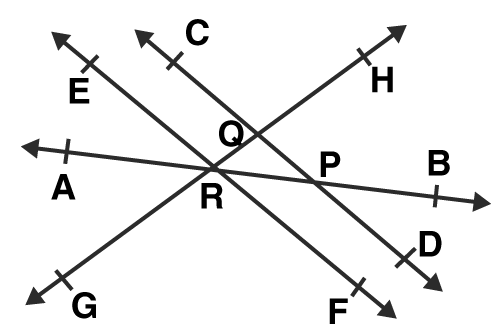Solution:

1.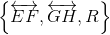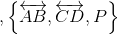2.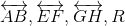3.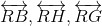4.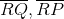5. From the given figure, name the following:

1. Three lines
2. One rectilinear figure
3. Four concurrent points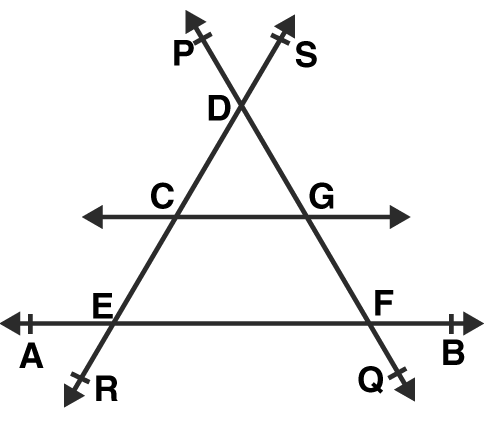Solution:

1. Three lines are line AB, line PQ and line RS
2. One rectilinear figure is CEFG
3. Four concurrent points are A, E, F and B.

6.

1. How many lines can be drawn to pass through a given point?
2. How many lines can be drawn to pass through two given points?
3. In how many points can the two lines at the most intersect?
4. If A, B, C are three collinear points, name all the line segments determined by them.

Solution:

1. Infinite number of lines can be drawn to pass through a given point.
2. Only one line can be drawn to pass through two given points.
3. Two lines at the most can intersect at one point only.
4.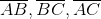7. Which of the following statements are true?

1. A line segment has no definite length.
2. A ray has no end point.
3. A line has a definite length.
4. A line
5. A ray
6. Two distinct points always determine a unique line.
7. Three lines are concurrent if they have a common point.
8. Two distinct lines cannot have more than one point in common.
9. Two intersecting lines cannot be both parallel to the same line.
10. Open half-line is the same thing as ray.
11. Two lines may intersect in two points.
12. Two lines are parallel only when they have no point in common.

Solution:

1. False. A line segment has definite length.
2. False. A ray has one end point.
3. False. A line has no definite length.
4. True.
5. False. BA and AB have different end points.
6. True.
7. True.
8. True.
9. True.
10. True.
11. False. Two lines intersect at only one point.
12. True.

8. In the given figure, L and M are the mid-points of AB and BC respectively.

1. If AB = BC, prove that AL = MC.
2. If BL = BM, prove that AB = BC.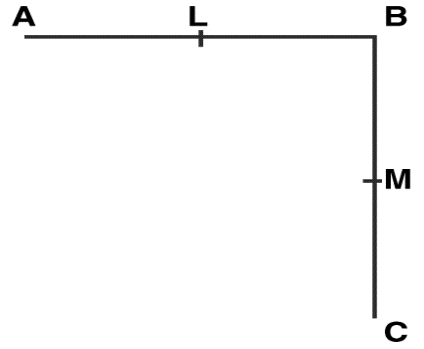Solution:

1. According to the diagramWe know that L is the midpoint of the line AB

So we get

AL = BL = 1/2 AB …….. (1)

We know that M is the midpoint of the line BC

So we get

BM = MC = 1/2 BC….. (2)

According to the question

It is given that

AB = BC

We can also write it as

1/2 AB = 1/2 BC

According to equation (1) and (2)

We can write it as

AL = MC

2. According to the diagramWe know that L is the midpoint of the line AB

So we get

AL = BL = 1/2 AB

The above equation can be written as

2AL = 2BL = AB ……. (3)

We also know that M is the midpoint of the line BC

BM = MC = 1/2 BC

The above equation can be written as

2BM = 2MC = BC ……. (4)

According to the question

It is given that

BL = BM

According to the equation (3) and (4)

We can write it as

AB = BC

### RS Aggarwal Solutions for Class 9 Maths Chapter 6: Introduction to Euclid’s Geometry

The Chapter 6, Introduction to Euclid’s Geometry, has 1 exercise with various axioms and postulates explained in brief and their applications in daily life. The topics which are mainly focused on in RS Aggarwal Solutions for Class 9 Chapter 6 are as follows:

• History
• Axioms and Postulates
• Euclid’s Axioms
• Euclid’s five postulates
• Incidence Axioms on Lines
• Number of Lines passing through two given points

### RS Aggarwal Solutions Class 9 Maths Chapter 6 – Introduction to Euclid’s Geometry

Students can refer RS Aggarwal Solutions for Class 9 Chapter 6 to have a good hold on the concepts and solve the problems that are difficult with ease. The brief and detailed solutions give a better chance to the students for understanding and learning concepts covered in this chapter.

These solutions can basically boost up the exam preparation of students for good results in the exams. Euclid’s Geometry has applications in various fields like Astronomy, Navigation, Surveying, Artistic Designs, Computer Aided Design, Architecture, Computer Aided Manufacturing and Classical Geometrical Problems.